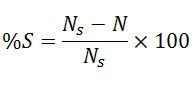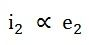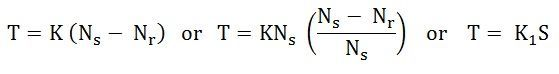# Slip Speed in an Induction Motor

Definition: The slip in an induction motor is the difference between the main flux speed and their rotor speed. The symbol S represents the slip. It is expressed by the percentage of synchronous speed. Mathematically, it is written asThe value of slip at full load varies from 6% in case of small motor and 2% in the large motor.

The induction motor never runs at synchronous speed. The speed of the rotor is always less than that of the synchronous speed. If the speed of the rotor is equal to the synchronous speed, no relative motion occurs between the stationary rotor conductors and the main field.

The no EMF induces in the rotor and zero current generates on the rotor conductors. The electromagnetic torque is also not induced. Thus, the speed of the rotor is always kept slightly less than the synchronous speed. The speed at which the induction motor work is known as the slip speed.

The difference between the synchronous speed and the actual speed of the rotor is known as the slip speed. In other words, the slip speed shows the relative speed of the rotor concerning the speed of the field.

The speed of the rotor is slightly less than the synchronous speed. Thus, the slip speed expresses the speed of the rotor relative to the field.

• If Ns is the synchronous speed in revolution per minute
• Nr is the actual rotor speed in revolution per minute.

The slip speed of the induction motor is given asThe fraction part of the synchronous speed is called the Per Unit Slip or Fractional Slip. The per unit slip is called the Slip. It is denoted by s.Therefore, the rotor speed is given by the equation shown below.Alternatively, if

• ns is the synchronous speed in revolution per second
• nr is the actual rotor speed in revolution per second.

Then,The percentage slip in revolution per second is given as shown below.The slip of the induction motor varies from 5 percent for small motors to 2 percent for large motors.

## Importance of Slip

Slip plays an essential role in Induction motor. As we know, the slip speed is the difference between the synchronous and rotor speed of the induction motor. The emf induces in the rotor because of the relative motion, or we can say the slip speed of the motor. So,The rotor current is directly proportional to the induced emf.The torque is directly proportional to the rotor current.Therefore,Hence, torque is directly proportional to slip.The above equation shows that the torque induces on the rotor is directly proportional to the slip of the induction motor. The high value of slip induces the emf in the rotor. This EMF develops the heavy torque on the rotor conductors.

The value of the slip is adjusted by considering the load on the motor. For full-load, the high value of torque is required. This can be achieved by increasing the amount of the slip and reducing the speed of the rotor. The slip of the motor is kept low when the induction motor is running at no-load. The small slip produces the small torque on the motor.

The value of the induction motor slip is adjusted according to the requirement of the driving torque at the normal working condition.